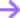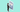# Data Analytics

Learn to analyse data and gain a solid foundation in statistics to better interpret your data for the purpose of enhancing your business making decisions.

### 612K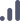All levels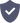Certified

## Recognized by -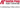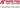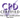### Overview

• Explore basic statistical skills including fundamental theories and terminology
• Discover R, data cleaning, data visualisation and packages in R that can be used for data analysis
• Use Excel for descriptive statistics
• Investigate Tableau as a data analysis platform
• Interpret and present results

Duration

Plus toolkits

Online

Certification

## Contents

module 1

### Professional Diploma in Data Analytics

+

-

1. Starting your Data Analyst Journey

Your journey to becoming a data analyst will start by understanding more about data and the analysis thereof. This lesson is geared to help you understand why data analysis is an important skill as well as where it can be used to enhance your business making decisions. Each one of our lessons is carefully balanced between theory and practical and in this lesson, you can expect to learn how to import and clean data using a variety of methods and tools. We will focus on logical checks to help guide you towards thinking about data in a more logical fashion.

2. Exploring Data

In this lesson, we will understand the data in a bit more detail. The aim is to assist you in understanding the different data types (such as categorical vs numerical) as well as understanding graphically represented data. You will also learn how to describe data (i.e. descriptive statistics) and how to implement descriptive statistics "features" in data.

3. Probability

As our journey continues, you will learn how to install the Data Analysis Toolpak together with some descriptive stats. We will touch briefly on the basics of probability (with specific reference to Bayes theorem) and delve into the details of mean and variance of random variables. This topic very neatly ties together a concept that we have covered (the mean) with one we are yet to cover (variance).

4. Distributing Data

Lesson 4 is all about distributing data. You will learn about the various data distributions (with reference to the central limit theorem) and understand how to use mean, median and standard deviation to know how your data is distributed. Lastly, we will also expand on skewness and kurtosis, so tune in if you are dying to know what this means for your data.

5. How Confident Are You In The Sample?

Being confident in your sample is important. This lesson will focus on understanding the difference between a sample and a population as well as when to use variance or standard deviation for each. We will also cover confidence intervals in more detail, and by the end of this lesson you will be well on your way to feeling more confident!

6. Hypothesizing About The Outcome

Understanding what a hypothesis is an important step in your journey. We will expand on what a null and alternative hypothesis is and explore the difference between a Type 1 and Type 2 error. This lesson will also include more information on the central limit theorem/ the law of large numbers to enlighten us on this topic.

7. Testing For Differences: Categorical Vars

Our penultimate lesson is focused on testing for differences (categorical vars). We will explore one sample tests, the difference between 2 means of 2 populations as well as Chi-square tests.

8. Testing For Differences: Numerical Vars

Finally, we wrap up with understanding testing for differences (numerical vars). In this lesson one sample tests, the difference between 2 means of 2 populations and T-tests will be covered and by the end of this lesson you will have a firm and complete understanding of the basics of data and data analysis. The journey does not stop here and in Module 2 you can expect more complex concepts and a deeper understanding of the topic.

module 2

### Intermediate in Data Analytics

+

-

1. Introduction to R

The objectives for this lesson are to add a new tool to your data analyst toolkit, called R. You will go through the basic steps of downloading and installing the tool and start exploring some of the packages that are available today in R. The lesson will conclude by introducing another common method to estimate population parameters, the maximum likelihood method.

2. Data Wrangling

The first topic will introduce the brilliant package, tidyverse. Thereafter, you will use R to reproduce some of the exploratory data analysis you have done with the Titanic dataset in Excel in Module 1. Today's lesson will conclude with an introduction to merging and joining datasets.

3. Introduction to Linear Regression

The first topic for this lesson will introduce linear regression. Thereafter you will dive deeper into understanding the concept of correlation. The lesson will end by going back to basics with vectors and factors in R.

4. Linear Regression ContinuedThis lesson will continue to broaden your understanding of linear regression and data frames. You will understand what it means for the model to fit the data well and gain some further insight into treating data in R. The lesson will end by exploring some basics surrounding dates values in R.
5. Dates and Times

In this lesson, we will continue to broaden our understanding of dealing with dates and times in R. Many datasets contain dates and times and we need to make the step of data handling dates and times as simple and effective in our data analytics arsenal as possible. Therefore, we will continue building on dates and times data wrangling throughout a large part of this lesson. We will end the lesson by introducing time series analysis concepts.

6. Time Series Analysis

This lesson will delve deeper into time series analysis. We will further discuss the concepts surrounding time series analysis that we introduced in the previous lesson and add some new concepts to that. Thereafter we will break down and understand some of the time series models a bit better. This lesson will end by looking at how we can apply these concepts learnt in R in a more practical sense.

7. Multiple Linear Regression

In this lesson, we will elaborate on the principles of multiple linear regression. We will elaborate on the assumptions that accompany multiple linear regression, how to simplify a multiple linear regression model, and problems that can occur when fitting a multiple linear regression model to the data.
Thereafter, we will discuss what happens if your model does not fit a linear trend well, in other words, if the data is non-linear.

The lesson will end by introducing logistic regression.

8. Introduction to Logistic Regression

Lesson 8 will elaborate on the introduction to logistic regression from lesson 7. We will better understand when to utilize this model and how to interpret the outcome. Thereafter we will elaborate on the model fit statistics we have briefly touched on in previous lessons, such as the AIC and BIC statistics. We will end the lesson by cementing in all the knowledge we have gained through module 2 with a practical demonstration.

## What makes us

### Stand out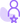### In-house experts

We don't outsource our content. Every course we make is our own and must meet our standards.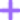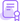### Shareable certificate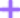### Learn about our certificates.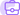### Careers service

Use what you've learnt to advance your career with our in-house careers service.